# ShineEternal的小书屋# ShineEternal的小书屋

https://blog.csdn.net/kkkksc03

### 数论小白都能看懂的平面凸包详解

posted on 2019-08-15 09:21:23 | under 日报 |

# 0.前言：

## 特别鸣谢：

• 画图：主要提供比较细的连线

• 画图3D：主要提供描点及文本框

# 1.引入：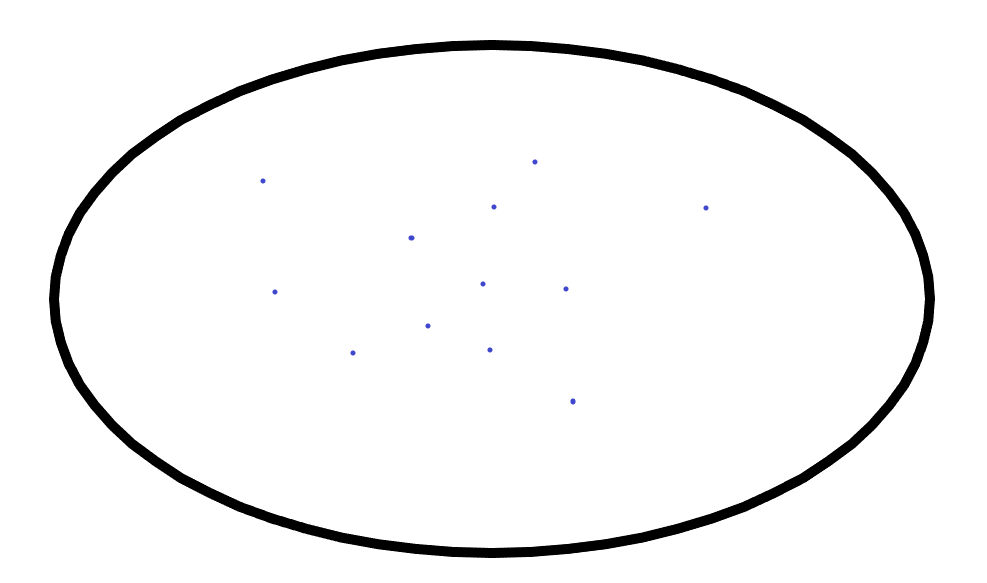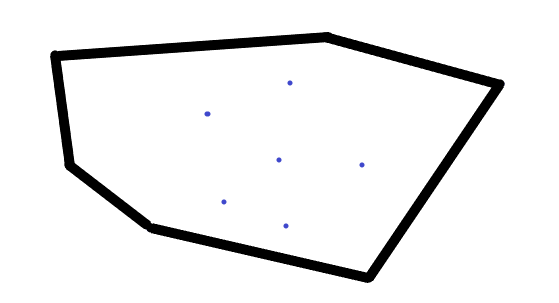# 2、斜率逼近法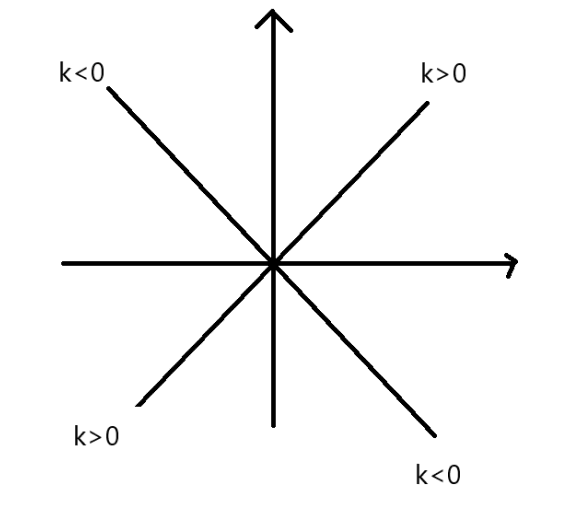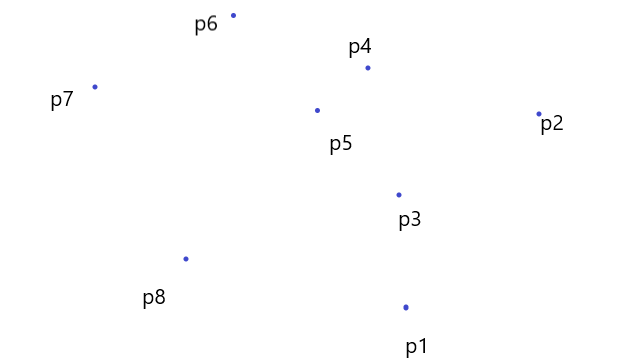（k是斜率）

• (1)首先在所有点中找出一个y值最小的点，记为 $P_1$
• (2)从 $P_1$ 出发，刚开始k=0，即为水平状态。然后按照上图的示意沿逆时针方向寻找。即开始在 $k>0$ 且 $(x_2>x1,y_2>y_1)$ 中找k最小的点 $P_2$ ，以此类推。

Q:如果过程中有多个点符合要求怎么办？

A:那么就取距离 $P_1$ 最远的点，因为这样能保证划定的范围最大。

• （3）从 $P_2$ 出发，用（2）的方法找 $P_3$

• (4)最后直到 $P_m=P_1$ 为止（已形成凸包）。

Q:为什么要刚开始找y值最小的点？

A:结合刚开始的小朋友拉绳子可知，我们在下面的绳子一定会被y值最小的小朋友挡住，即他一定在凸包上，于是就以他为基准来操作。

Q:万一最后没有一个 $P_m$ 使得 $P_m=P_1$ 呢？

A:易证必有，平面凸包总是存在的。

n为所有小朋友的数量，m为舍己为人的小朋友的数量。

# 3、Jarvis算法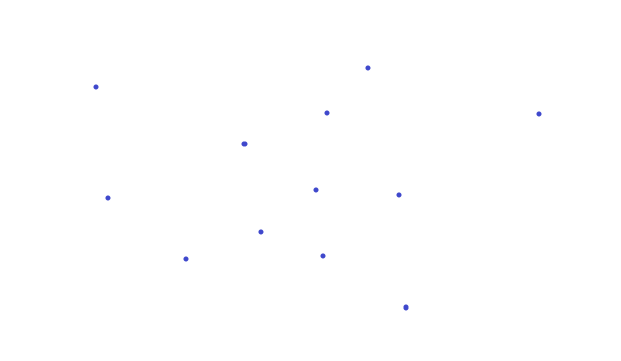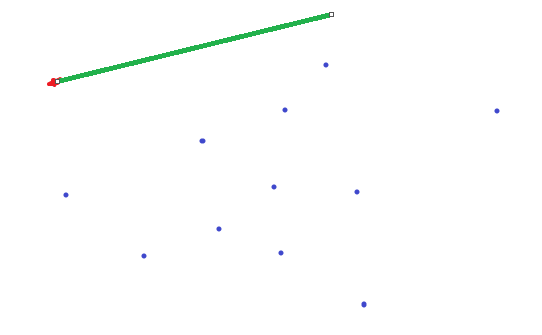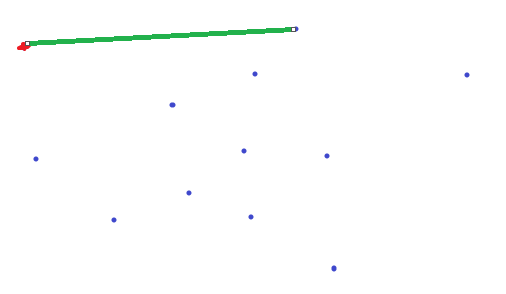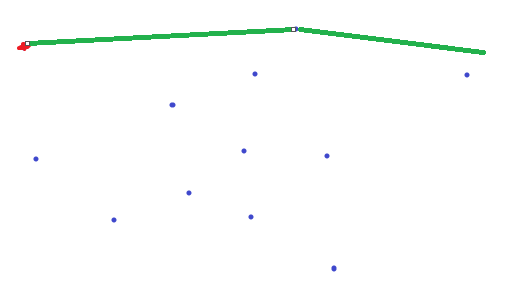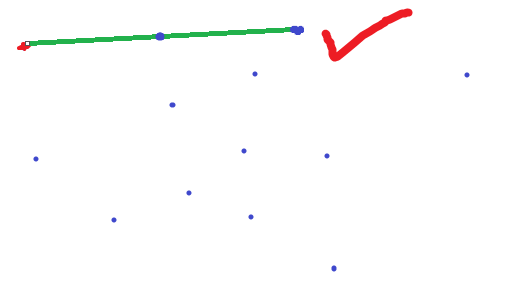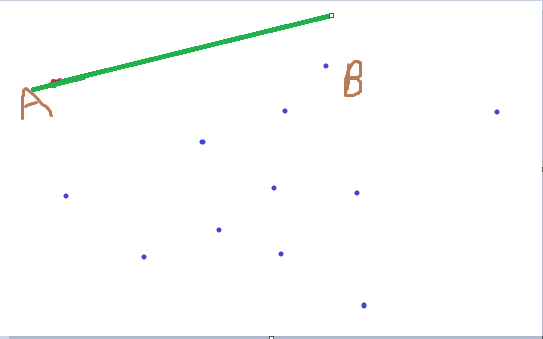• 首先找到一条直线 $l$ 过其中一点A，使得所有其他的点都在 $l$ 的同一侧。

• 让直线 $l$ 以A为轴点沿顺时针或逆时针方向旋转，直到抡到除A以外的一点B

• 重复以上操作，直到l碰到A点。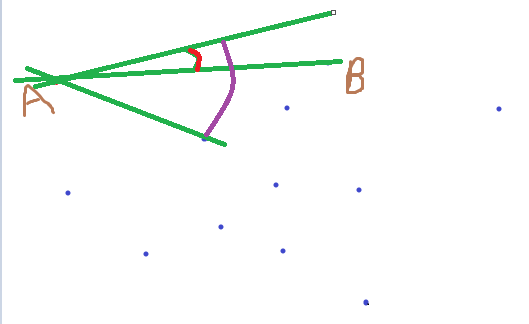$if(\vec {AP}\times \vec {AP_i})z>0$

$else$

$if(\vec {AP}\times \vec {AP_i})z=0$

# 4、Graham算法

Graham扫描算法维护一个凸壳 通过不断在凸壳中加入新的点和去除影响凸性的点 最后形成凸包

• 排序

• 扫描

## （1）排序

• 我们还是选择一个y值最小（如有相同选x最小）的点，记为 $P_1$

• 剩下的点集中按照极角的大小逆时针排序，然后编号为 $P_2$ ~ $P_m$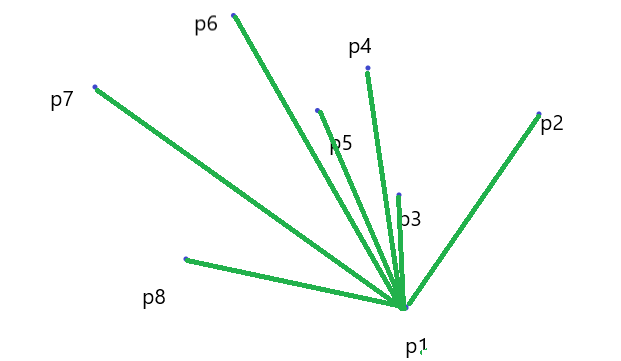• 我们按照排序结束时的顺序枚举每一个点，依次连线，这里可以使用一个栈来存储，每次入栈，如果即将入栈的元素与栈顶两个元素所构成了一个类似于凹壳的东西，那么显然处于顶点的那个点一定不在这个点集的凸包上，而他正好在栈顶，所以把它弹出栈，新点入栈。

（ps:下列解说中右转左转等是指以上一条连线为铅垂线，新的连线偏移的方位）p4出现了右转现象，那么我们就把顶点p3舍去，在检查p2的性质，合格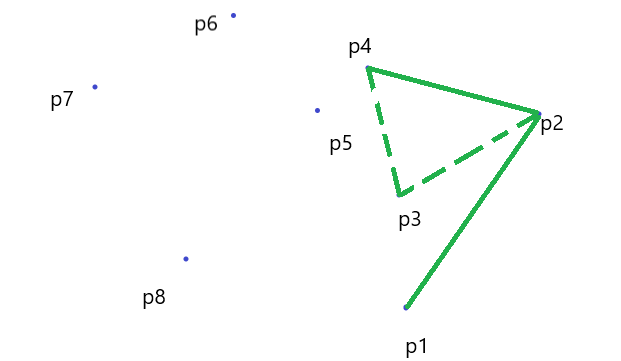p5一切正常，入栈。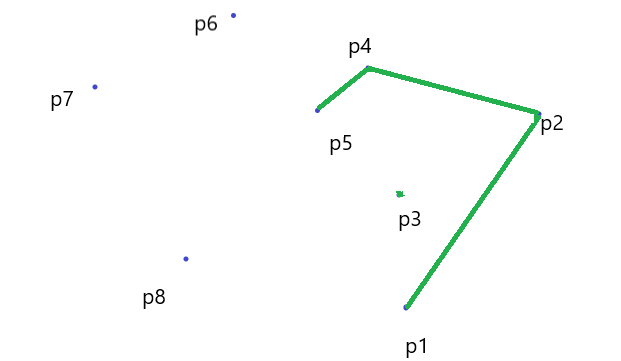p6这里就要复杂一些

• 首先他往右转，于是将p5弹出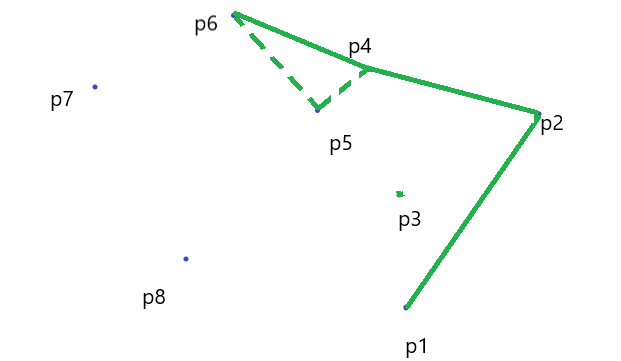• 又发现他相对于 $P_2P_4$ 向右转，于是将p4弹出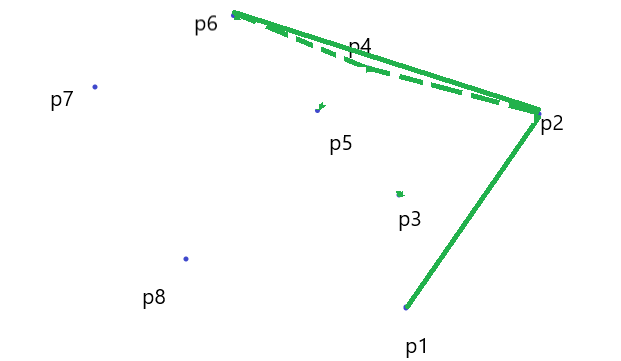p7一切正常（左转），入栈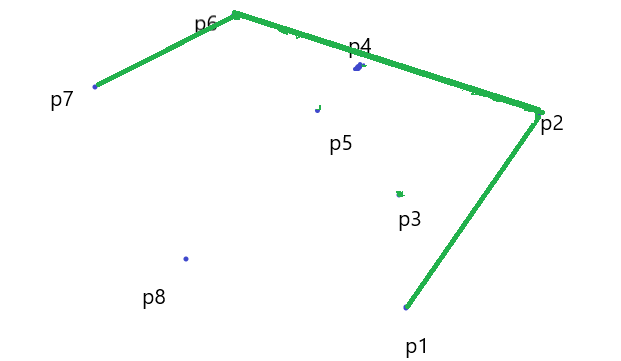p8一切正常（左转），入栈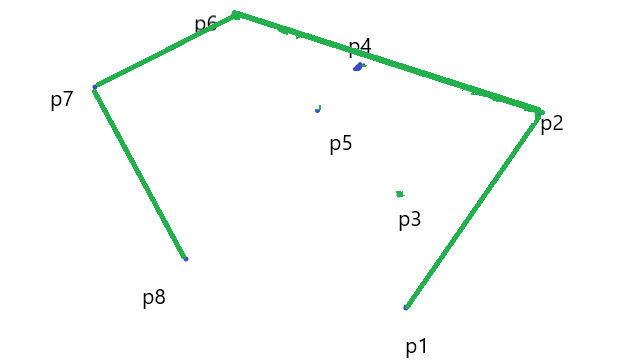P2742 【模板】二维凸包 / [USACO5.1]圈奶牛Fencing the Cows

### code：

#include<iostream>
#include<algorithm>
#include<cstdio>
#include<cmath>
using namespace std;
int n;
struct ben
{
double x,y;
}p,s;
double check(ben a1,ben a2,ben b1,ben b2)//检查叉积是否大于0，如果是a就逆时针转到b
{
return (a2.x-a1.x)*(b2.y-b1.y)-(b2.x-b1.x)*(a2.y-a1.y);
}
double d(ben p1,ben p2)//两点间距离。。。
{
return sqrt((p2.y-p1.y)*(p2.y-p1.y)+(p2.x-p1.x)*(p2.x-p1.x));
}
bool cmp(ben p1,ben p2)//排序函数，这个函数别写错了，要不然功亏一篑
{
double tmp=check(p,p1,p,p2);
if(tmp>0)
return 1;
if(tmp==0&&d(p,p1)<d(p,p2))
return 1;
return 0;
}
int main()
{

scanf("%d",&n);
double mid;
for(int i=1;i<=n;i++)
{
scanf("%lf%lf",&p[i].x,&p[i].y);
if(i!=1&&p[i].y<p.y)//这是是去重
{
mid=p.y;p.y=p[i].y;p[i].y=mid;
mid=p.x;p.x=p[i].x;p[i].x=mid;
}
}
sort(p+2,p+1+n,cmp);//系统快排
s=p;//最低点一定在凸包里
int cnt=1;
for(int i=2;i<=n;i++)
{
while(cnt>1&&check(s[cnt-1],s[cnt],s[cnt],p[i])<=0) //判断前面的会不会被踢走，如果被踢走那么出栈
cnt--;
cnt++;
s[cnt]=p[i];
}
s[cnt+1]=p;//最后一个点回到凸包起点
double ans=0;
for(int i=1;i<=cnt;i++)
ans+=d(s[i],s[i+1]);//然后s里存好了凸包序列，只需要把两两距离累加就行
printf("%.2lf\n",ans);
return 0;
}

# 4、例题：

## 信用卡凸包

P3829 [SHOI2012]信用卡凸包

### 题意简叙：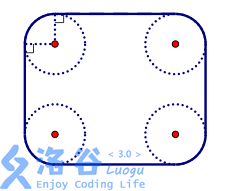### 分析：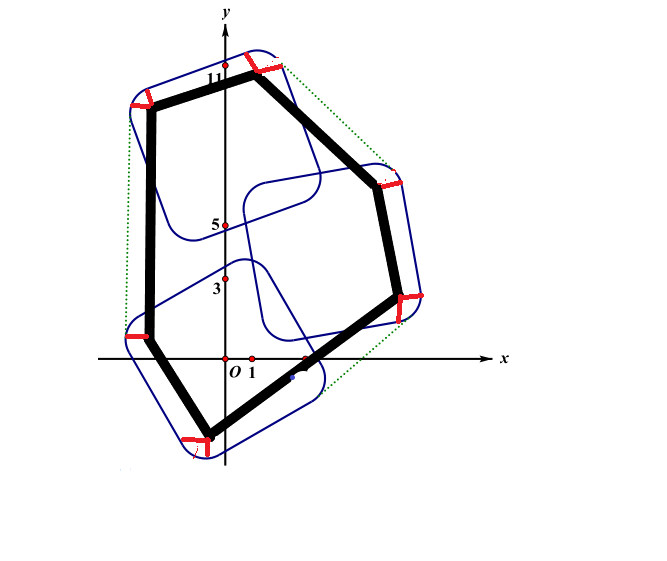# 5、Andrew算法

（评论区里一个大连提出了这个补充，于是添加一下QAQ）

### 主体思路：

• 按照x优先的顺序排序（坐标从小到大）

• 从第一个点开始遍历，如果下一个点在栈顶的两个元素所连成直线的左边，那么就入栈；

• 否则如果在右边，说明凸包有更优的方案，上次的点出栈，并直到新点在栈顶两个点所在的直线的左边为止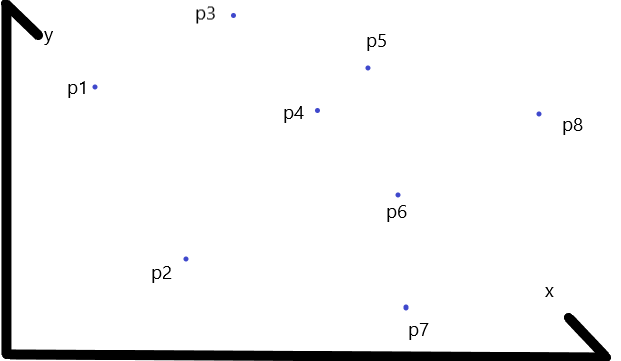• 首先p1一定是在凸包顶点上的。

• p2可能在，也可能不在，等着之后判断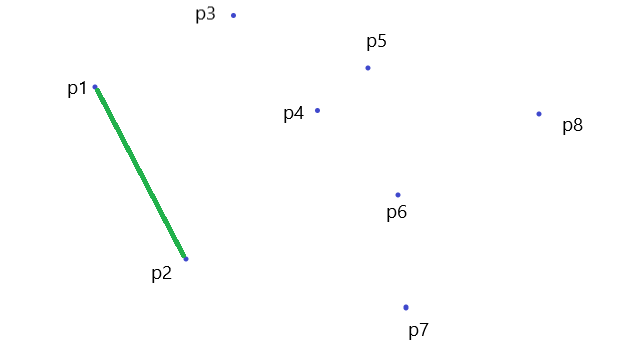p3偏左，入栈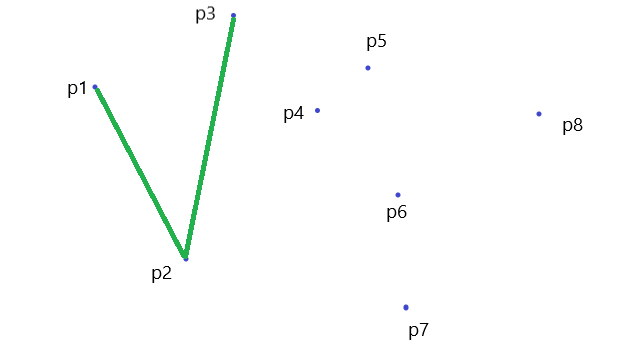p4和p3连线，发现偏右，于是p3出栈，检查p2正常，于是p4再入栈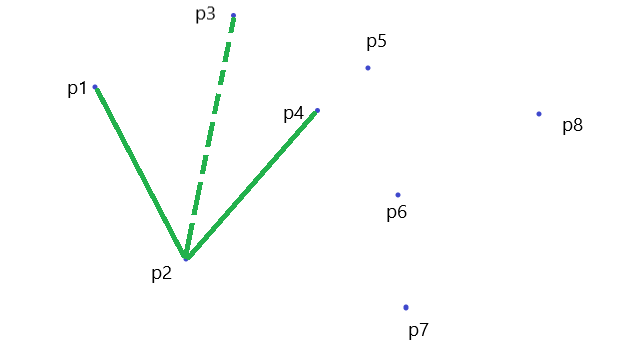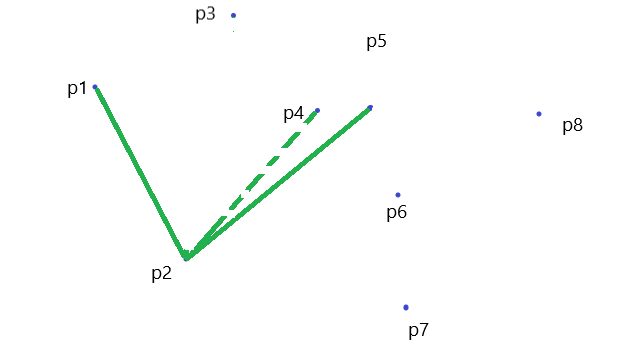p5出栈，p6出栈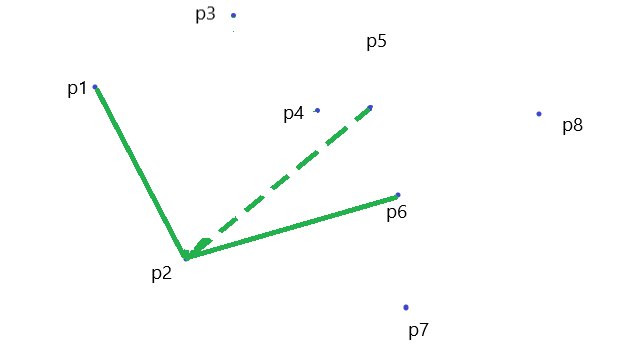p6出栈，p7入栈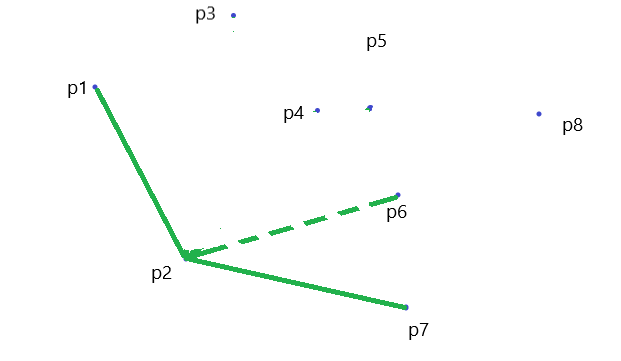p8一马平川的入栈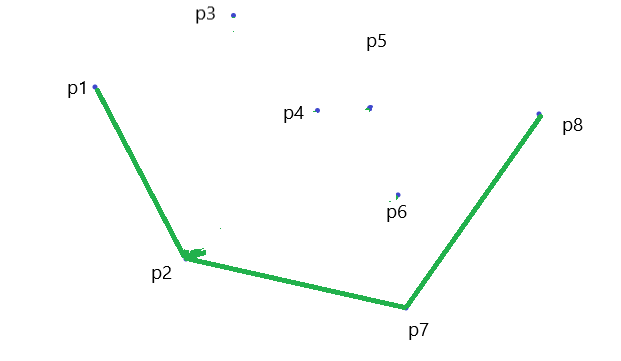### 然后我们惊讶的发现：• 如果你按照本文的方法行动，那就是一个下凸包

### 那下面就揭晓答案：

• 先走到p6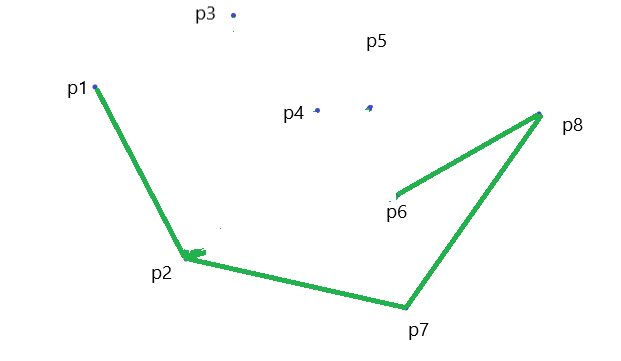• p6出栈，p5入栈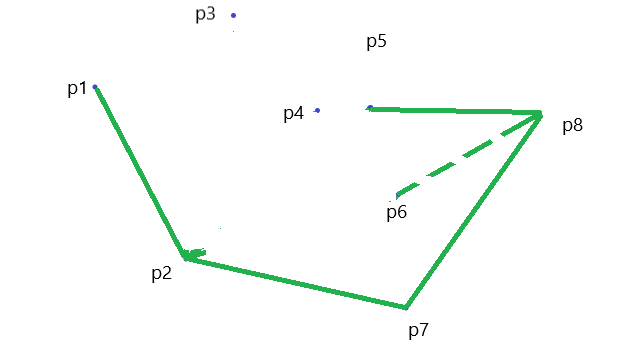• 这里可能画的不是很清楚，其实是不共线的

• 如果共线也好处理，直接取最长的哇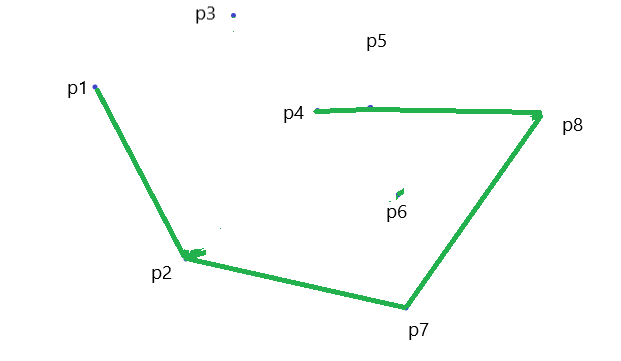• 他首先把p4给请出去了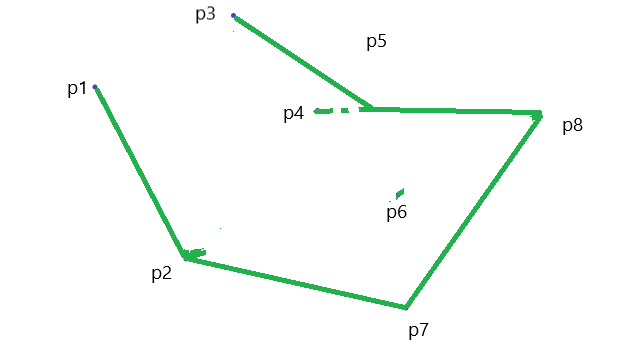• 然后又把p5给请出去了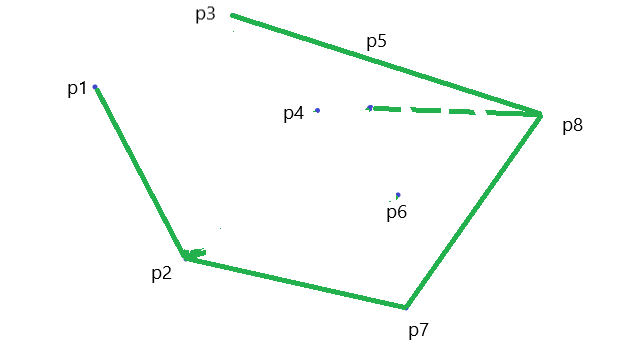## code:

double Cross(vec A, vec B)
{
return A.x*B.y-A.y*B.x; //A->B左转为正
}
double Side(vec a, vec b, vec p) //次栈顶元素a，栈顶元素b，新增点p
{
vec A=vec(b.x-a.x,b.y-a.y); //向量ab
vec B=vec(p.x-a.x,p.y-a.y); //向量ap
return Cross(A,B);
}
int Andrew(int top)
{
sort(p+1,p+n+1);
if(n<3)
{
printf("-1\n");
return;
}
st=p,st=p;
top=1;
for (int i=3;i<=n;i++)//从p1开始的下凸包
{
while(top&&Side(st[top-1],st[top],p[i])<=0)
top--;
st[++top]=p[i];
}
st[++top]=p[n-1];
for(int i=n-2;i>=1;i--)//从pn开始的上凸包
{
while(top&&Side(st[top-1],st[top],p[i])<=0)
top--;
st[++top]=p[i];
}
}